Set which counts the number of times a values are added to it and assigns them a unique positive index. : Set « Collections Data Structure « Java

Set which counts the number of times a values are added to it and assigns them a unique positive index.

```
//package opennlp.tools.util;

import java.io.FileOutputStream;
import java.io.FileWriter;
import java.io.IOException;
import java.io.OutputStreamWriter;
import java.io.PrintWriter;
import java.util.Collection;
import java.util.HashMap;
import java.util.Iterator;
import java.util.Map;
import java.util.Set;

/**
* Set which counts the number of times a values are added to it and assigns them a unique positive index.
* These value can be accessed with the #getCount() or #getIndex() method.
*/

public class CountedNumberedSet extends NumberedSet {
private Map cset;
private int max;

/**
* Creates a new counted set.
*/
public CountedNumberedSet() {
cset = new HashMap();
max=1;
}

/** Creates a new counted set of the specified initial size.
* @param size The initial size of this set.
*/
public CountedNumberedSet(int size) {
cset = new HashMap(size);
max = 1;
}

int[] nums = (int[]) cset.get(o);
if ( nums == null ) {
cset.put(o, new int[] {1,max++});
return true;
}
else {
nums++;
return false;
}
}

/**
* Reduces the count associated with this object by 1.  If this causes the count
* to become 0, then the object is removed form the set.
* @param o The object whose count is being reduced.
*/
public void subtract(Object o) {
int[] count = (int[]) cset.get(o);
if ( count != null ) {
count--;
if (count <= 0) {
cset.remove(o);
}
}
}

/**
* Assigns the specified object the specified count in the set.
* @param o The object to be added or updated in the set.
* @param c The count of the specified object.
*/
public void setCount(Object o,int c) {
int[] nums = (int[]) cset.get(o);
if (nums != null) {
nums = c;
}
else {
cset.put(o,new int[]{c,1});
}
}

/**
* Return the count of the specified object.
* @param o the object whose count needs to be determined.
* @return the count of the specified object.
*/
public int getCount(Object o) {
int[] nums = (int[]) cset.get(o);
if (nums == null ) {
return 0;
}
else {
return nums;
}
}

/**
* Returns the index for the specified key or -1 if specified value is not contain in this set.
* @param key The key to be checked.
* @return the index for the specified value or -1 if specified value is not contain in this set
*/
public int getIndex(Object key) {
int[] nums = (int[]) cset.get(key);
if (nums == null) {
return -1;
}
else {
return nums;
}
}

public void write(String fileName,int countCutoff) {
write(fileName,countCutoff," ");
}

public void write(String fileName,int countCutoff,String delim) {
write(fileName,countCutoff,delim,null);
}

public void write(String fileName,int countCutoff,String delim,String encoding) {
PrintWriter out = null;
try{
if (encoding != null) {
out = new PrintWriter(new OutputStreamWriter(new FileOutputStream(fileName),encoding));
}
else {
out = new PrintWriter(new FileWriter(fileName));
}

for (Iterator e = cset.keySet().iterator();  e.hasNext();) {
Object key = e.next();
int count = this.getCount(key);
if ( count >= countCutoff ) {
out.println(count + delim + key);
}
}
out.close();
}
catch (IOException e) {
System.err.println(e);
}
}

boolean changed =  false;
for (Iterator ci = c.iterator();ci.hasNext();) {
}
return changed;
}

public void clear() {
cset.clear();
}

public boolean contains(Object o) {
return cset.keySet().contains(o);
}

public boolean containsAll(Collection c) {
return cset.keySet().containsAll(c);
}

public boolean isEmpty() {
return cset.isEmpty();
}

public Iterator iterator() {
return cset.keySet().iterator();
}

public boolean remove(Object o) {
return cset.remove(o) != null;
}

public boolean removeAll(Collection c) {
boolean changed =false;
for (Iterator ki = cset.keySet().iterator();ki.hasNext();) {
changed = changed || cset.remove(ki.next()) != null;
}
return changed;
}

public boolean retainAll(Collection c) {
boolean changed = false;
for (Iterator ki = cset.keySet().iterator();ki.hasNext();) {
Object key = ki.next();
if (!c.contains(key)) {
cset.remove(key);
changed = true;
}
}
return changed;
}

public int size() {
return cset.size();
}

public Object[] toArray() {
return cset.keySet().toArray();
}

public Object[] toArray(Object[] arg0) {
return cset.keySet().toArray(arg0);
}
}
class NumberedSet implements Set {

private int max;
private Map nset;

public NumberedSet() {
super();
nset = new HashMap();
max=1;
}

public NumberedSet(int size) {
super();
nset = new HashMap(size);
max=1;
}

/**
* Returns the index for the specified key or -1 if specified value is not contain in this set.
* @param key The key to be checked.
* @return the index for the specified value or -1 if specified value is not contain in this set
*/
public int getIndex(Object key) {
Integer i = (Integer) nset.get(key);
if (i == null) {
return -1;
}
else {
return i.intValue();
}
}

public void setIndex(Object key, int index) {
nset.put(key, Integer.valueOf(index));
}

public int size() {
return nset.size();
}

public boolean isEmpty() {
return nset.isEmpty();
}

public boolean contains(Object o) {
return nset.containsKey(o);
}

public Iterator iterator() {
return nset.keySet().iterator();
}

public Object[] toArray() {
return nset.keySet().toArray();
}

public Object[] toArray(Object[] arg0) {
return nset.keySet().toArray(arg0);
}

if (!nset.containsKey(arg0)) {
nset.put(arg0, Integer.valueOf(max++));
return true;
}
return false;
}

public boolean remove(Object o) {
return nset.remove(o) != null;
}

public boolean containsAll(Collection c) {
return nset.keySet().containsAll(c);
}

boolean changed =  false;
for (Iterator ci = c.iterator();ci.hasNext();) {
}
return changed;
}

public boolean retainAll(Collection c) {
boolean changed = false;
for (Iterator ki = nset.keySet().iterator();ki.hasNext();) {
Object key = ki.next();
if (!c.contains(key)) {
nset.remove(key);
changed = true;
}
}
return changed;
}

public boolean removeAll(Collection c) {
boolean changed =false;
for (Iterator ki = nset.keySet().iterator();ki.hasNext();) {
changed = changed || nset.remove(ki.next()) != null;
}
return changed;
}

public void clear() {
nset.clear();
}

}

```

Related examples in the same category

 1 Set, HashSet and TreeSet 2 Things you can do with Sets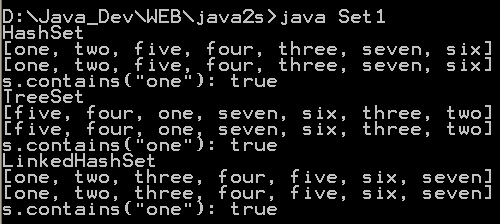3 Set operations: union, intersection, difference, symmetric difference, is subset, is superset 4 Set implementation that use == instead of equals() 5 Set that compares object by identity rather than equality 6 Set union and intersection 7 Set with values iterated in insertion order. 8 Putting your own type in a Set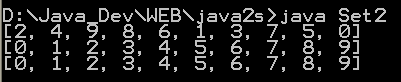9 Use set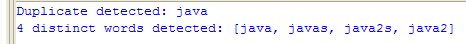10 Another Set demo 11 Set subtraction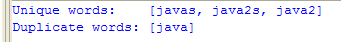12 Working with HashSet and TreeSet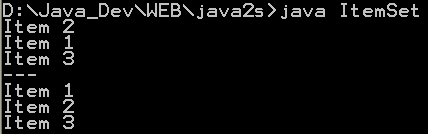13 TreeSet Demo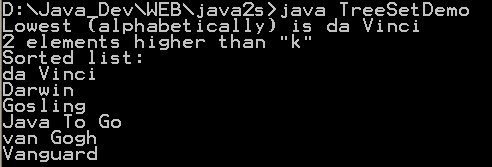14 Show the union and intersection of two sets 15 Demonstrate the Set interface 16 Array Set extends AbstractSet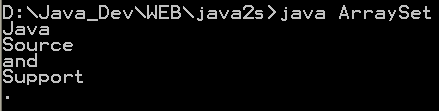17 Sync Test 18 Set Copy 19 Set and TreeSet 20 Tail 21 What you can do with a TreeSet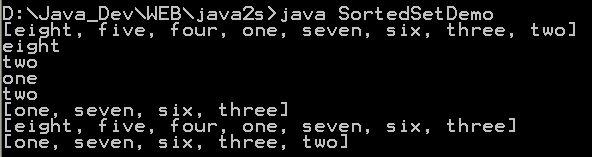22 Remove all elements from a set 23 Copy all the elements from set2 to set1 (set1 += set2), set1 becomes the union of set1 and set2 24 Remove all the elements in set1 from set2 (set1 -= set2), set1 becomes the asymmetric difference of set1 and set2 25 Get the intersection of set1 and set2, set1 becomes the intersection of set1 and set2 26 Extend AbstractSet to Create Simple Set 27 Int Set 28 One Item Set 29 Small sets whose elements are known to be unique by construction 30 List Set implements Set 31 Converts a char array to a Set 32 Converts a string to a Set 33 Implements the Set interface, backed by a ConcurrentHashMap instance 34 An IdentitySet that uses reference-equality instead of object-equality 35 An implementation of the java.util.Stack based on an ArrayList instead of a Vector, so it is not synchronized to protect against multi-threaded access. 36 A thin wrapper around a List transforming it into a modifiable Set. 37 A thread-safe Set that manages canonical objects 38 This program uses a set to print all unique words in System.in 39 Indexed Set 40 An ObjectToSet provides a java.util.Map from arbitrary objects to objects of class java.util.Set. 41 Sorted Multi Set 42 Fixed Size Sorted Set 43 Set operations 44 A NumberedSet is a generic container of Objects where each element is identified by an integer id. 45 Set which counts the number of times a values are added to it. 46 Indexed Set 47 A set acts like array. 48 Implements a Bloom filter. Which, as you may not know, is a space-efficient structure for storing a set. 49 Implementation of disjoint-set data structure 50 Call it an unordered list or a multiset, this collection is defined by oxymorons